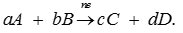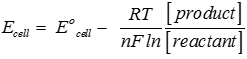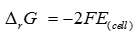# Electrochemistry - Online Test

Q1. Electrochemistry is the study of production of electricity from
Explaination / Solution:

Electrochemistry is branch of chemistry that deals with production of electricity from a chemical reaction or carrying out a non spontaneous reaction using electricity.

Q2. Galvanic or a voltaic cell converts the chemical energy liberated during a redox reaction to
Explaination / Solution:

Galvanic cells convert chemical energy to electrical energy.

Q3. Electrolytic cell is a device for using electrical energy to carry
Explaination / Solution:

Electrolytic cell converts non spontaneous chemical reaction to electrical energy using electricity.

Q4. Fundamental difference between an electrolytic cell and the galvanic cell is that in an electrolytic cell free energy
Explaination / Solution:

In electrolytic cell electrical energy is converted into chemical energy.

Q5. Standard electrode potential are measured with the concentrations of all the species involved in a half-cell is
Explaination / Solution:

Under standard condition concentration of every species is taken as 1.

Q6. standard hydrogen electrode operated under standard conditions of 1 atm H2 pressure, 25°C, and pH = 0 has a cell potential of
Explaination / Solution:

Standard electrode potential of hydrogen electrode is taken as 0V.

Q7. For a general electrochemical reaction of the type :Nernst equation can be written as:
Explaination / Solution:Q8. Relationship between equilibrium constant of the reaction and standard potential of the cell in which that reaction takes place is
Explaination / Solution:

At equilibrium E cell becomes zero .

Q9. The reversible work done by a galvanic cell is equal to decrease in its Gibbs energy and therefore, if the emf of the cell is E and nF is the amount of charge passed and ΔrG is the Gibbs energy of the reaction, then
Explaination / Solution:Q10. Molar conductivity denoted by the symbol Λm is related to the conductivity of the solution by the equation (κis the conductivity and c the concentration)
Explaination / Solution:

Molar conductivity is specific conductivity of a solution having 1 mole of electrolyte dissolved per litre of solution.